# SSC Quantitative Aptitude Practice Questions (Day-4)

Dear Aspirants, Here we have given the Important SSC CGL Exams 2018 Practice Test Papers. Candidates, those who are preparing for SSC CGL Exams 2018 can practice these questions to get more confidence to Crack SSC CGL Exams 2018 Examination.

[WpProQuiz 4081]

1) What is the unit digit of the sum of the first 110 natural numbers?

a) 4

b) 6

c) 5

d) 0

2) How many 10 digit positive number are there?

a) 9 × 109

b) 9 × 1010

c) 10 × 1010

d) 11 × 108

3) What is the value of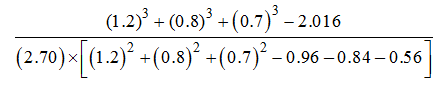a) 1/4

b) 1/2

c) 1

d) 2

4) What is the unit digit of (317)409 × 419543 × 424628×302816?

a) 2

b) 4

c) 6

d) 8

5) N is the largest two digit number which when divided by 3,4 and6 leaves the remainder 1, 2 and 4 respectively what will be the remainder when ‘N’ is divided by 7?

a) 4

b) 3

c) 0

d) 1

6) Which one of the following is true?

a) √11 > √7 > ∜45

b) √7 > ∛11 > ∜45

c) √7 > ∜45 >∛11

d) ∜45 > √7 > ∛11

7) What is the value of 113 + 123 + 133 + ………….. + 303?

a) 213000

b) 213200

c) 213400

d) 213600

8) Find the value of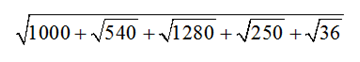a) 100

b) 34

c) 32

d) 54

9) If p= 7+43 and PQ = 1, Then what is the value of 1/ p2 + 1/q2?

a) 194

b) 196

c) 200

d) 204

10) If (x+y)/z = 6/5, (y+z)/x = 9/2, Then what is the value of x + z/y?

a) 9/5

b) 11/7

c) 7/11

d) 13/4

11) If α and β are roots of equation x2 – x + 1, which equation will have roots α2 and β2?

a) x2 + 2x + 1

b) x2 + x + 1

c) x2 – x + 1

d) x2 – 2x + 1

12) If 3x+ 5y + 7z = 56 and 9x+8y +21z =126, what is the value of y?

a) 4

b) 2

c) 6

d) 5

13) Price of 4 mango, 6 oranges and 9 guavas is Rs 305. Price of 3 mango, 4 oranges and 2 guava is rupees 145. What is the cost of 5 mango, 8 oranges and 16 guavas in rupees?

a) 415

b) 465

c) 440

d) Cannot be determined

14) How many 3 cm radius solid spheres can be get after melting 18cm diameter solid spheres?

a) 216

b) 6

c) 27

d) 36

15) What is the Value of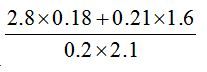a) 2

b) 1

c) 3

d) 1.5

Sum of first N natural numbers = n(n+1)/2 = 110 (110 + 1)/2

= (110× 111)/2 = 55 × 111 = 6105

One digit Positive No = 9 x 100

Two digit Positive No = 9 x 101

Three digit Positive No = 9 x 102

10 digit Positive No= 9 x 109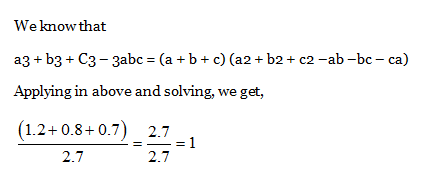Last digit (317)409 = 1

Last digit (419)543 = 9

Last digit (424)628= 6

Last digit (302)816 = 2

Last digit of Multiple 8 is Answer

Minimum No. 10

LCM of 3, 4, 6 = 12

No. Near to 99,

=> 10 + (12 ×7)

H when 94 divided by 7 = 3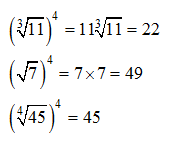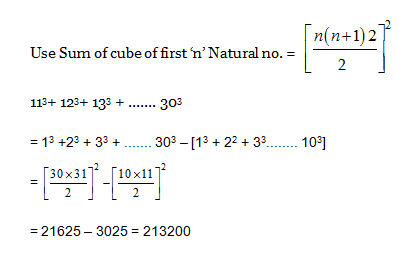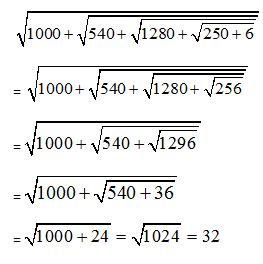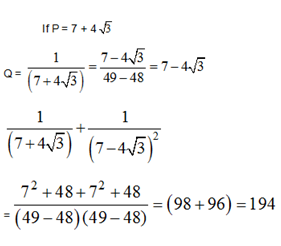(x+y)/z = 6/5                         (y + z)/x = 9/2

x + y = 6                                 x = 2

2 + y = 6                                 y = 4

z = 5

x + z/y = 2 + (5/4) = 13/4

If α & β are root, of each x2-x+1 then, sum of roots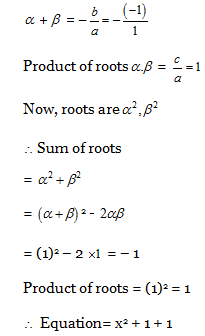3x+ 5y + 7z = 56 —— (1)

9x+8y +21z =126 —– (2)

Multiplying eq. (i) × 3

9x + 15y + 21z = 168

Subtracting eq. from (i)

9x + 15y + 21z = 168

(-)9x+8y +21z =126

________________________

7y = 42

y = 6

4M + 6 O + 9 G = 305           – (i)

3 M + 4 O + 2 G = 145           – (ii)

Hence, Eq (i) × 2 – Eq. (ii)

5 M + 8.0 + 16 G = (305 × 2 – 145) = 465

Volume of sphere = 4/3 πr3

No of sphere = (4/3 πR3)/ (4/3 πr3) = 93 / 33

= (9×9×9)/ (3×3×3) = 27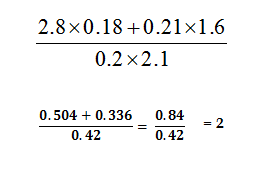*****************************